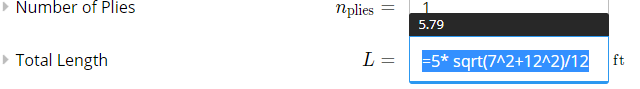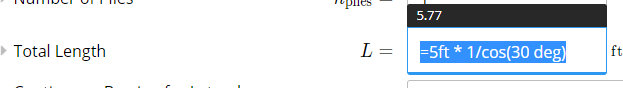# How to Design a Sloped or Angled Member

To calculate a sloped member in ClearCalcs, you need to use user formulas to adjust lengths and support locations by the rise/run or degrees of the given slope. In this way, you can still enter the length of a rafter from the plan drawing and adjust it in ClearCalcs using the simple formulas below.

Using Rise/Run

The formula for 7:12in rise over run is:

Member Length * SquareRoot(Rise^2+Run^2)/Run
=5ft * sqrt(7^2+12^2)/12


Sub in the rise required rise and member length

Example 1: 5ft beam with rise of 7:12 inchesUsing Degrees

You can also adjust the length of a member based on degree slope:

Member Length * 1/cos(theta)
=5ft * 1/cos(30deg)


Example 2: 5ft beam with slope of 30 degrees - N.B. You need to specify either 'deg' or 'degree' units for the degrees so it calculates correctlyUsing a Slope Factor

You can also multiply the plan length by the appropriate slope factor.